# MISSION BANKING 2023 Quantitative Aptitude Quiz- 15

## MISSION BANKING 2023 Quantitative Aptitude Quiz

Quantitative Aptitude is the most significant part of almost all competitive exams. Candidates appear to be having difficulty with Quantitative Aptitude Questions, so they need to practice more. Even if the portion is difficult, if you practice enough questions and learn the basic concepts, you can surely do well. In this article, we have come up with the MISSION BANKING 2023 Quantitative Aptitude Quiz to enhance your preparation. This MISSION BANKING 2023 Quantitative Aptitude Quiz will assist you to cope with the tough competition. This MISSION BANKING 2023 Quantitative Aptitude Quiz is available to you at no cost. Through this MISSION BANKING 2023 Quantitative Aptitude Quiz, you will learn formulas and short tricks to solve the Quantitative Aptitude Questions. Candidates must solve this MISSION BANKING 2023 Quantitative Aptitude Quiz to improve their exam.

1. I. 5x+2y=31
II. 3x+7y=36

(a) if x<y

(b) if x> y

(c) if x≤ y

(d) if x≥ y

(e) if x= y or no relation can be established(a) if x<y

(b) if x> y

(c) if x≤ y

(d) if x≥ y

(e) if x= y or no relation can be established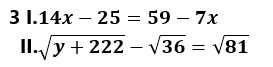(a) if x<y

(b) if x> y

(c) if x≤ y

(d) if x≥ y

(e) if x= y or no relation can be established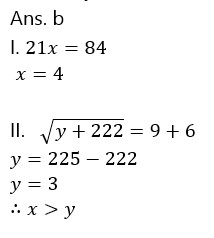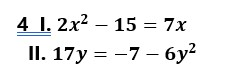(a) if x<y

(b) if x> y

(c) if x≤ y

(d) if x≥ y

(e) if x= y or no relation can be established(a) if x<y

(b) if x> y

(c) if x≤ y

(d) if x≥ y

(e) if x= y or no relation can be established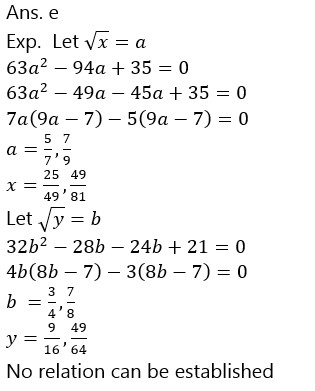1. A is 50% more than B, C is 2/3 rd of A and D is 60% more than C. Now if each of A, B, C and D is increased by 10%. Then find D is what percent of B after the increase.

(a) 150%

(b) 160%

(c) 175%

(d) 180%

(e) 170%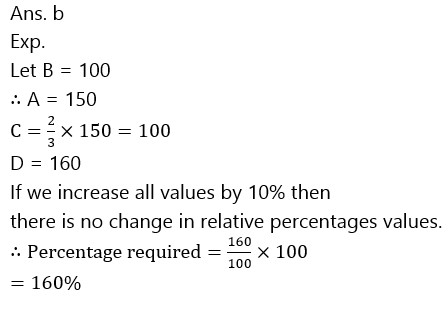1. A man invests a part of Rs. 10000 at 5% and the remainder at 6%. The 5% investment yields annually Rs. 76.50 more than the part invested at 6%. The amount invested at 6% is

(a) Rs. 3600

(b) Rs. 3850

(c) Rs. 3500

(d) Rs. 40000

(e) None of these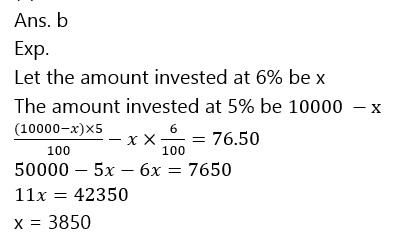1. The batting average for 30 innings of a cricket player is 40 runs. His highest score exceeds his lowest score by 100 runs. If these two innings are not included, the average of the remaining 28 innings is 38 runs. The lowest score of the player is :

(a) 15

(b) 18

(c) 20

(d) 12

(e) None of these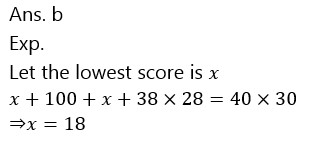1. If selling price of 12 mangoes equals to cost price of 25 oranges and selling price of 10 oranges is equal to cost price of 9 Mangoes, then find the profit % earn by selling 1 Mango and 1 Orange if cost price of 3 Mangoes is equal to cost price of 5 oranges.

(a) 38.55%

(b) 36.525%

(c) 36.475%

(d) 34.375%

(e) 32.425%1. The distance between two cities A and B is 330km. A train starts from A at 8 am. and travels towards B at 60 km/hr. Another train starts from B at 9 am. and travels towards A at 75 km/hr. At what time do they meet?

(a) 10 am.

(b) 10 : 30 am.

(c) 11 am.

(d) 11 : 30 am.

(e) None of these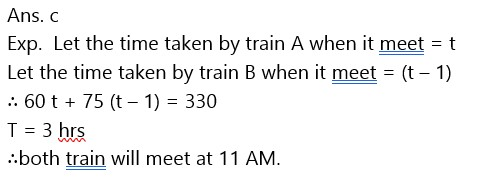### 2023 Preparation Kit PDF

#### Most important PDF’s for Bank, SSC, Railway and Other Government Exam : Download PDF Now

AATMA-NIRBHAR Series- Static GK/Awareness Practice Ebook PDF Get PDF here
The Banking Awareness 500 MCQs E-book| Bilingual (Hindi + English) Get PDF here
AATMA-NIRBHAR Series- Banking Awareness Practice Ebook PDF Get PDF here
Computer Awareness Capsule 2.O Get PDF here
AATMA-NIRBHAR Series Quantitative Aptitude Topic-Wise PDF 2020 Get PDF here
Memory Based Puzzle E-book | 2016-19 Exams Covered Get PDF here
Caselet Data Interpretation 200 Questions Get PDF here
Puzzle & Seating Arrangement E-Book for BANK PO MAINS (Vol-1) Get PDF here
ARITHMETIC DATA INTERPRETATION 2.O E-book Get PDF here

3